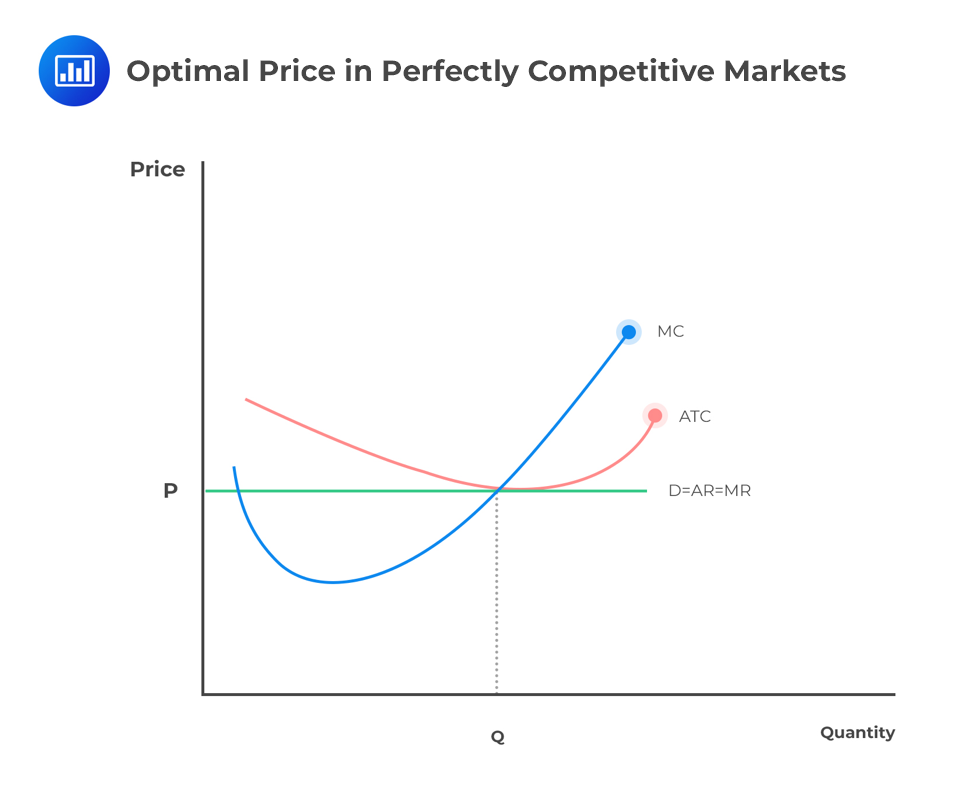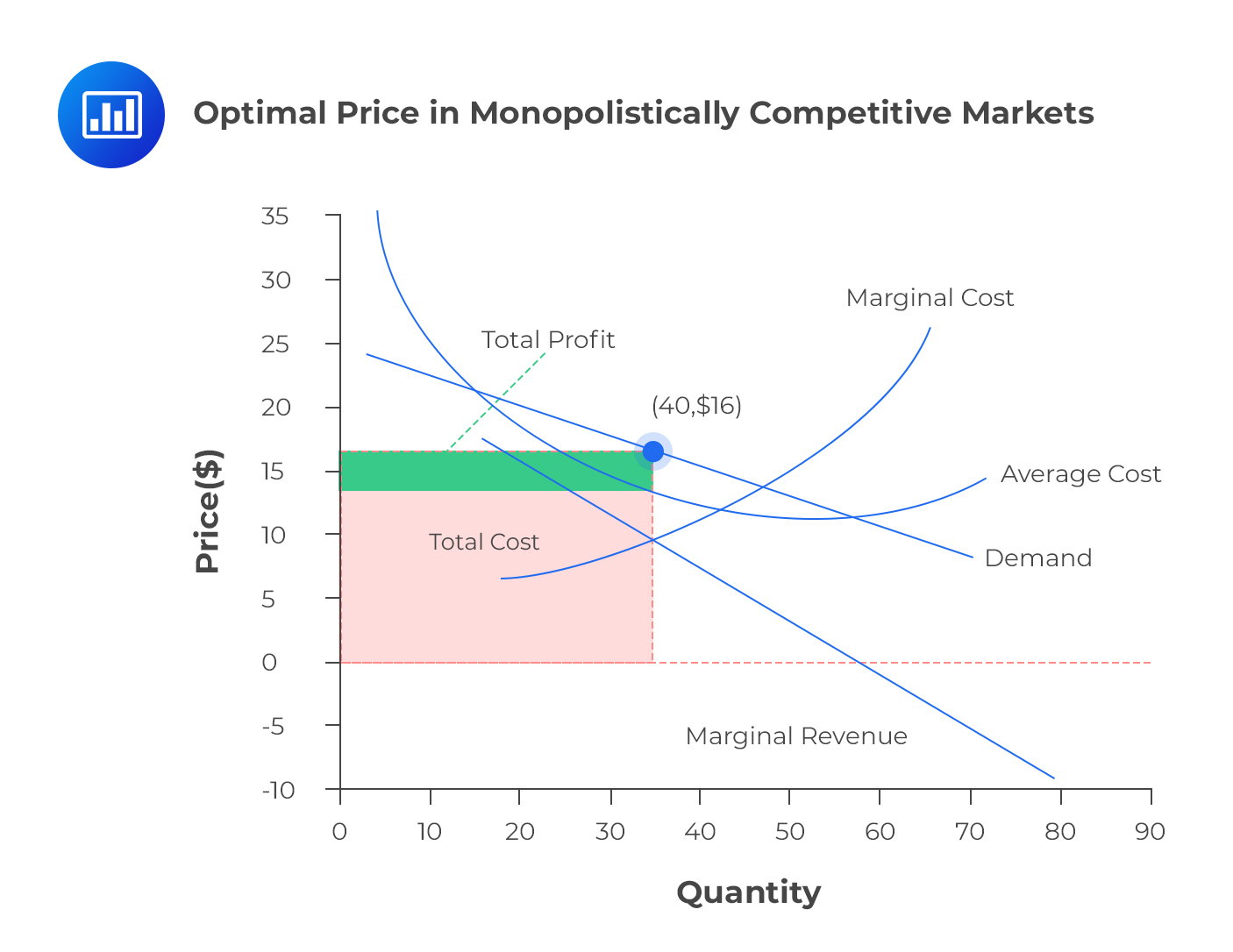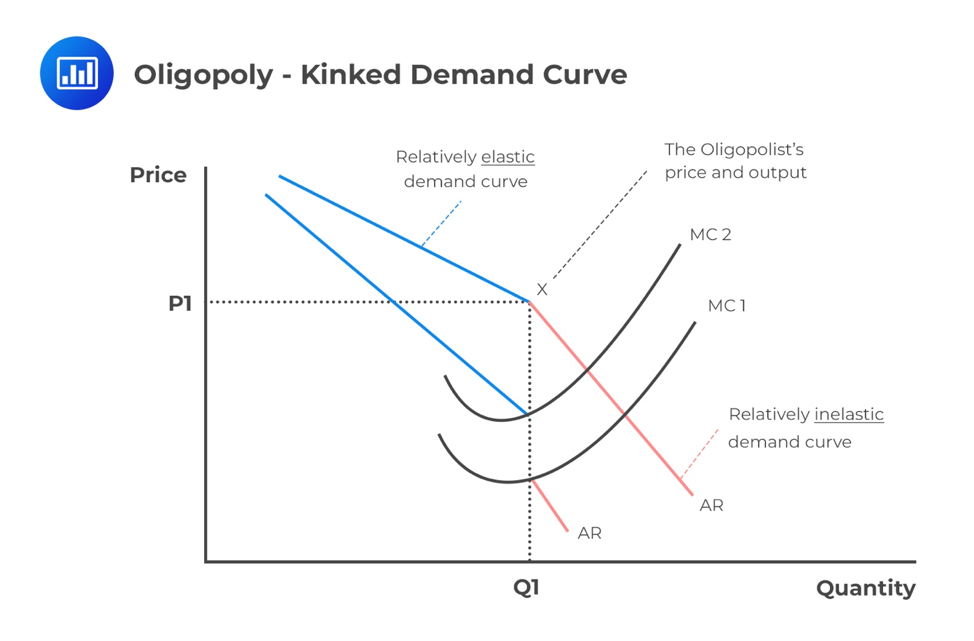Limited Time Offer: Save 10% on all 2022 Premium Study Packages with promo code: BLOG10# Optimal Price and Output Levels Under Different Market Structures

An optimal price can be defined as the price at which a seller can make the highest profit possible; that is, the seller’s price is maximized. The rule of marginal output postulates that profit is maximized by producing an output, whereby the marginal cost (MC) of the last unit produced is exactly equal to the marginal revenue (MR). Simply put, MC=MR.

## Optimal Price and Output in Perfectly Competitive Markets

Under perfect competition, there are many firms in the market. If one firm decides to increase production, the market equilibrium price won’t be highly affected. Conversely, if a single firm changes the market price at equilibrium, then the market won’t be in perfect competition. Therefore, each firm faces a demand curve that is horizontal at equilibrium.

In perfect competition, any profit-maximizing producer has a market price equal to its marginal cost (P=MC).### Example of Optimal Price and Output in Perfectly Competitive Markets

If the price function P = 20 – Q, and MC = 5 + 2Q, calculate the profit-maximizing price and output.

#### Solution

The profit is maximized when:

$$P = MC \Rightarrow 20 – Q = 5 + 2Q$$

$$\therefore 3Q=15 \Rightarrow Q=5$$

Substituting Q into the price function:

$$P = 20 – 5 = 15$$

The profit-maximizing price and output are 15 and 5, respectively.

## Optimal Price and Output in Monopolistically  Competitive Markets

In this market structure, the short-run profit-maximizing choice occurs at the point where marginal revenue is equal to marginal cost (MR=MC). Total revenue (TR) is a product of price and quantity:

$$TR = P\times Q$$

The average cost incurred in producing Q units of a product is taken as C. Therefore, the total cost (TC) is calculated as the product of average cost and total quantity. That is,

$$TC = C\times Q$$

The economic profit is the difference between TR and TC. We denote the economic profit by π. Then,

$$\pi= TR-TC$$## Optimal Price and Output in Oligopoly Markets

There is no well-defined optimal price and optimal output in this market structure. In fact, this has already been discussed in the previous learning objective about the supply function of an oligopolistic market. There exist many firms that form an oligopoly. These firms all have their own pricing models. When there exists a kinked curve, the optimal price is taken at the point of the kink. However, the kinked demand curve can’t explain the cause of that kink.In the case of a dominant firm, the output level is vital in determining the optimal price. This occurs at the point where MR = MC. The profit-maximizing price is thus calculated by that segment of the demand function that the dominant firm faces.

Moreover, when we have a Cournot model (in which companies compete on the amount of output they will produce), every firm assumes that the other firms cannot alter their price levels of output with respect to the dominant firm. Their optimum price is still at MR = MC.

In a Nash equilibrium (no participant can gain by a unilateral change of strategy), firms react to their own circumstances and maximize their own profits. This continues until each firm is independently stable.

## Optimal Price and Output in Monopoly Market

The combination of output and price that maximizes profit occurs at the elastic portion of the demand curve. This is because MR and MC will always intersect at the point where MR is positive. In this case, demand is elastic when MC = MR. The relationship between MR and price elasticity,$$E_p$$is

$$MR=P[1-\frac{1}{E_p}]$$

In a monopoly, MR = MC thus:

$$MR=P[1-\frac{1}{E_p}]=MC$$

A firm informed of its cost structure and its price elasticity($$E_p$$) can use this relationship to work out its profit-maximizing price.

### Example of Optimal Price and Output in Monopoly Market

The marginal cost(MC) of a production company is  \$100. From the past market analysis, the price elasticity was approximated to be 1.5. Calculate the optimal price.

#### Solution

Using the equation,

$$P[1-\frac{1}{E_p}]=MC$$

$$\Rightarrow P[1-\frac{1}{1.5}]=100 \therefore P=300$$

### Question

A firm that is operating in a monopolistically competitive market environment maximizes its profits when:

1. the average cost is minimized.
2. marginal revenue equals average cost.
3. marginal revenue equals marginal cost.

Solution

The correct answer is C.

The firm will maximize its profit when the level of output is such that, the marginal revenue equals marginal cost. In other words, it will produce the quantity such that MR=MC.

A and B are incorrect. From the graph given below, we can clearly see that it’s neither the point where average cost is minimized nor the point where marginal revenue equals average cost.Shop CFA® Exam Prep

Offered by AnalystPrepLevel I
Level II
Level III
All Three Levels
Featured Shop FRM® Exam PrepFRM Part I
FRM Part II
FRM Part I & Part II
Learn with Us

Subscribe to our newsletter and keep up with the latest and greatest tips for success
Shop Actuarial Exams PrepExam P (Probability)
Exam FM (Financial Mathematics)
Exams P & FM
Shop GMAT® Exam PrepComplete CourseSergio Torrico
2021-07-23
Excelente para el FRM 2 Escribo esta revisión en español para los hispanohablantes, soy de Bolivia, y utilicé AnalystPrep para dudas y consultas sobre mi preparación para el FRM nivel 2 (lo tomé una sola vez y aprobé muy bien), siempre tuve un soporte claro, directo y rápido, el material sale rápido cuando hay cambios en el temario de GARP, y los ejercicios y exámenes son muy útiles para practicar.diana
2021-07-17
So helpful. I have been using the videos to prepare for the CFA Level II exam. The videos signpost the reading contents, explain the concepts and provide additional context for specific concepts. The fun light-hearted analogies are also a welcome break to some very dry content. I usually watch the videos before going into more in-depth reading and they are a good way to avoid being overwhelmed by the sheer volume of content when you look at the readings.Kriti Dhawan
2021-07-16
A great curriculum provider. James sir explains the concept so well that rather than memorising it, you tend to intuitively understand and absorb them. Thank you ! Grateful I saw this at the right time for my CFA prep.nikhil kumar
2021-06-28
Very well explained and gives a great insight about topics in a very short time. Glad to have found Professor Forjan's lectures.Marwan
2021-06-22
Great support throughout the course by the team, did not feel neglectedBenjamin anonymous
2021-05-10
I loved using AnalystPrep for FRM. QBank is huge, videos are great. Would recommend to a friendDaniel Glyn
2021-03-24
I have finished my FRM1 thanks to AnalystPrep. And now using AnalystPrep for my FRM2 preparation. Professor Forjan is brilliant. He gives such good explanations and analogies. And more than anything makes learning fun. A big thank you to Analystprep and Professor Forjan. 5 stars all the way!michael walshe
2021-03-18
Professor James' videos are excellent for understanding the underlying theories behind financial engineering / financial analysis. The AnalystPrep videos were better than any of the others that I searched through on YouTube for providing a clear explanation of some concepts, such as Portfolio theory, CAPM, and Arbitrage Pricing theory. Watching these cleared up many of the unclarities I had in my head. Highly recommended.